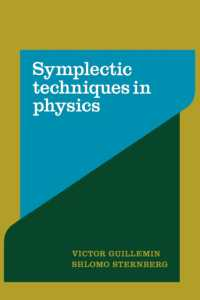### Symplectic Techniques in Physics （Reprint）

• 提携先の海外書籍取次会社に在庫がございます。通常2週間で発送いたします。
重要ご説明事項
1. 納期遅延や、ご入手不能となる場合が若干ございます。
2. 複数冊ご注文の場合、分割発送となる場合がございます。
3. 美品のご指定は承りかねます。
• ≪洋書のご注文につきまして≫ 「海外取次在庫あり」および「国内仕入れ先からお取り寄せいたします」表示の商品でも、納期の目安期間内にお届けできないことがございます。あらかじめご了承ください。

• 製本 Paperback:紙装版/ペーパーバック版
• 言語 ENG
• 商品コード 9780521389907
• DDC分類 519

### Full Description

Symplectic geometry is very useful for clearly and concisely formulating problems in classical physics and also for understanding the link between classical problems and their quantum counterparts. It is thus a subject of interest to both mathematicians and physicists, though they have approached the subject from different view points. This is the first book that attempts to reconcile these approaches. The authors use the uncluttered, coordinate-free approach to symplectic geometry and classical mechanics that has been developed by mathematicians over the course of the last thirty years, but at the same time apply the apparatus to a great number of concrete problems. In the first chapter, the authors provide an elementary introduction to symplectic geometry and explain the key concepts and results in a way accessible to physicists and mathematicians. The remainder of the book is devoted to the detailed analysis and study of the ideas discussed in Chapter 1. Some of the themes emphasized in the book include the pivotal role of completely integrable systems, the importance of symmetries, analogies between classical dynamics and optics, the importance of symplectic tools in classical variational theory, symplectic features of classical field theories, and the principle of general covariance. This work can be used as a textbook for graduate courses, but the depth of coverage and the wealth of information and application means that it will be of continuing interest to, and of lasting significance for mathematicians and mathematically minded physicists.

### Table of Contents

`Preface1. Introduction2. The geometry of the moment map3. Motion in a Yang-Mills field and theprinciple of general covariance4. Complete integrability5. Contractions of symplectic homogeneous spacesReferencesIndex.`